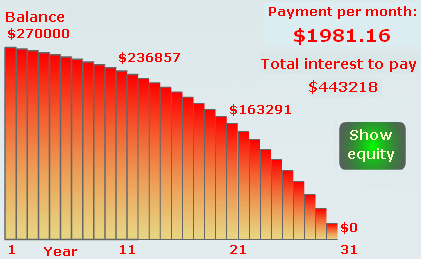Search IntMath
Close

# Housing mortgage rounding error

By Murray Bourne, 22 Sep 2006

Matt, one of the users of Interactive Mathematics said...

Hey there i was using your calculator for mortgage and there seems to be some sort of mistake in the code, i was testing mine against yours and getting different answers then i looked up at what the monthly payment is supposed to be in your math equation and i noticed it was .16 instead of the .92 that your get in your calculator just so you know! so your other numbers are off by about 200!

My response to Matt was...

Hi Matt. Thanks a lot for your feedback - much appreciated. Yep - there is a problem in there - maybe it was a rounding error in the Flash file, since the .16 at the top is correct. But as I said on the page, banks will find a way to charge you more, so whatever we calculate, we can guarantee the amount they charge will be higher!

I do like this sort of feedback. It means that users are trying to figure out the mathematics and are willing to let me know of a problem.

Anyway, it was a rounding error as anticipated. I had rounded the monthly interest amount to the nearest cent and then used that amount later in the code. Leaving it at full accuracy fixed the problem.

I also took it as an opportunity to tidy up the Flash interactive.

Here is a screen shot of what we are talking about:Be the first to comment below.

### Comment Preview

HTML: You can use simple tags like <b>, <a href="...">, etc.

To enter math, you can can either:

1. Use simple calculator-like input in the following format (surround your math in backticks, or qq on tablet or phone):
a^2 = sqrt(b^2 + c^2)
(See more on ASCIIMath syntax); or
2. Use simple LaTeX in the following format. Surround your math with $$ and $$.
$$\int g dx = \sqrt{\frac{a}{b}}$$
(This is standard simple LaTeX.)

NOTE: You can mix both types of math entry in your comment.